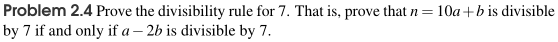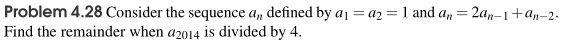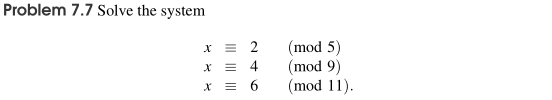# Math Challenge II-A Textbooks

Please see the sections below for answer keys, topics covered, and sample problems for each of the MC II-A textbooks.

### Algebra

The answer key for MC II-A Algebra is available by clicking here.

#### Chapter Topics

1. Factoring Fundamentals
2. Quadratics
3. Problem Solving with Quadratics
4. Advanced Factoring
5. Solving Equations with Fractions
6. Solving Equations with Absolute Values
7. Solving Equations with Radicals
8. Polynomials
9. Problem Solving Polynomials
10. Math Contest Review

#### Sample Questions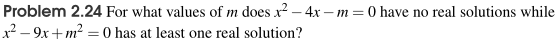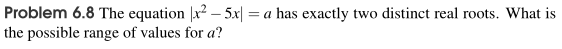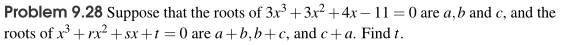### Geometry

The answer key for MC II-A Geometry is available by clicking here.

#### Chapter Topics

1. Special Angles
2. Special Angles II
3. Analytical Geometry
4. Centers
5. Areas
6. Areas II
7. Circles
8. Circles II
9. Solid Geometry
10. Math Contest Review

#### Sample Questions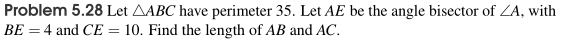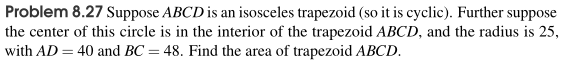### Combinatorics

The answer key for MC II-A Combinatorics is available by clicking here.

#### Chapter Topics

1. Counting Fundamentals
2. Grouping and Spacing
3. Stars and Bars
4. Sets and PIE
5. Advanced Counting
6. Combinatorial Identities
7. Recurrence Relations
8. Probability Fundamentals
9. Advanced Probability
10. Math Contest Review

#### Sample Questions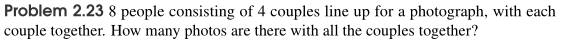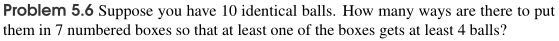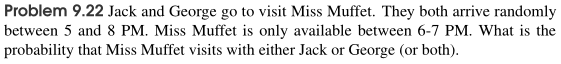### Number Theory

The answer key for MC II-A Number Theory is available by clicking here.

#### Chapter Topics

1. Place Values and Number Bases
2. Divisibility, Divisors, and Multiples
3. Problem Solving with Prime Numbers
4. Modular Arithmetic
5. Problem Solving with Modular Arithmetic
6. Fundamental Theorems in Modular Arithmetic
7. Advanced Theorems in Modular Arithmetic
8. Diophantine Equations
9. Solving Equations with the Floor Function
10. Math Contest Review

#### Sample Questions